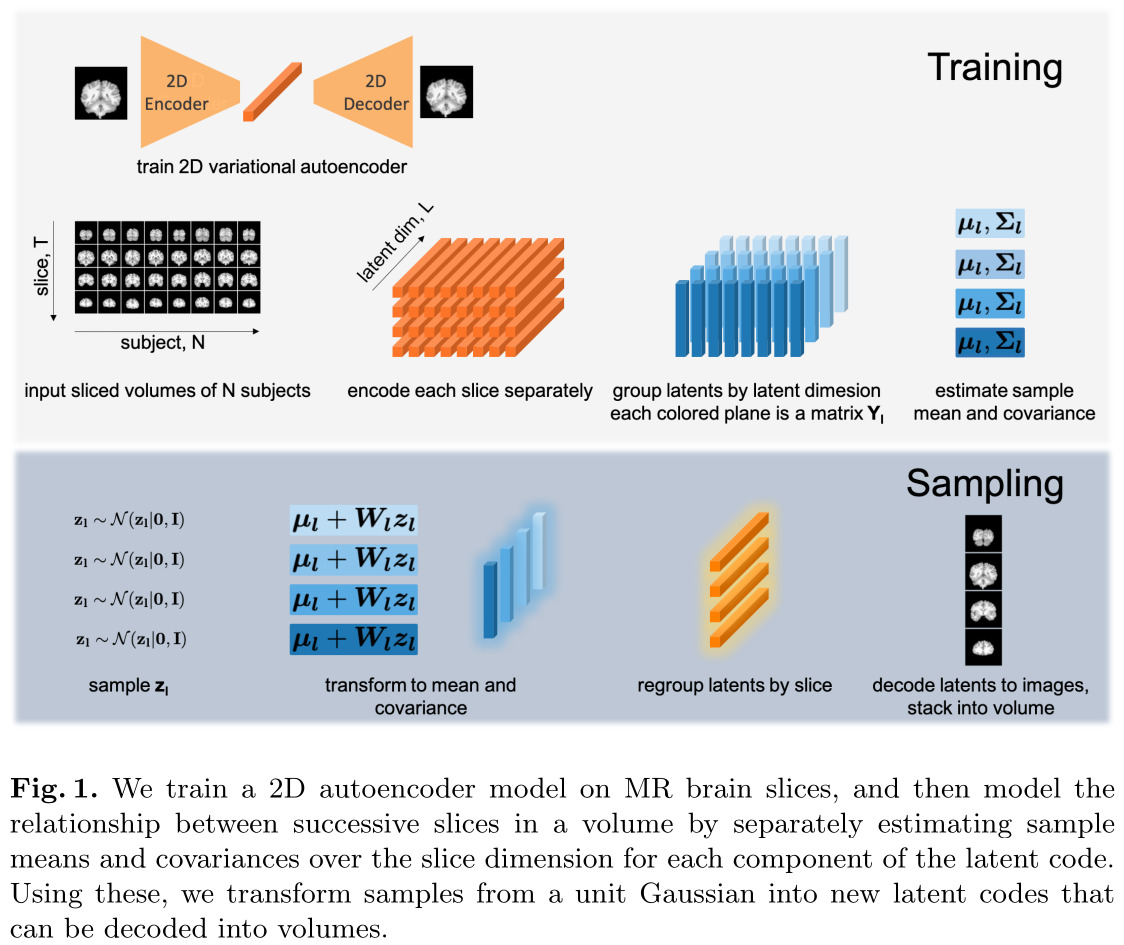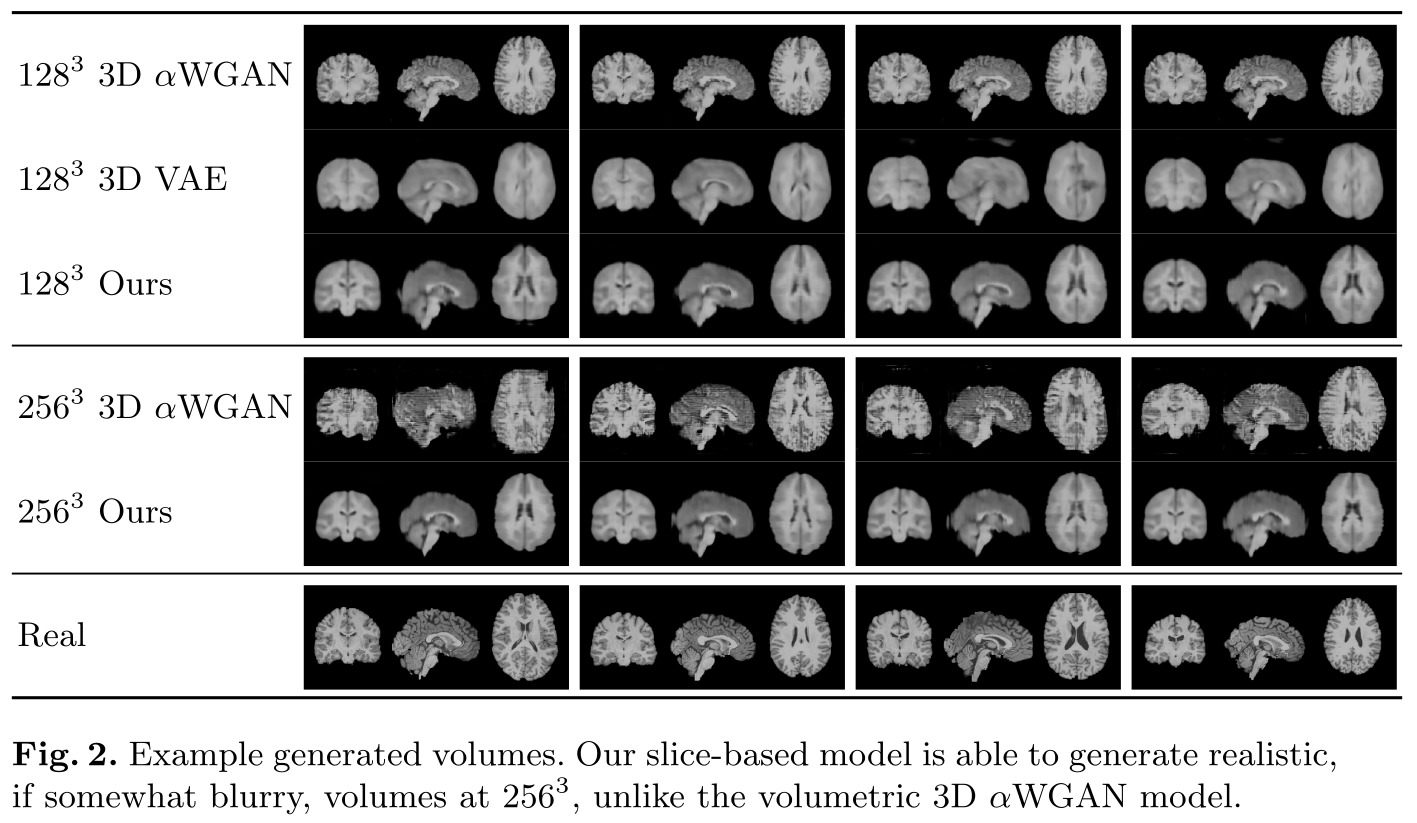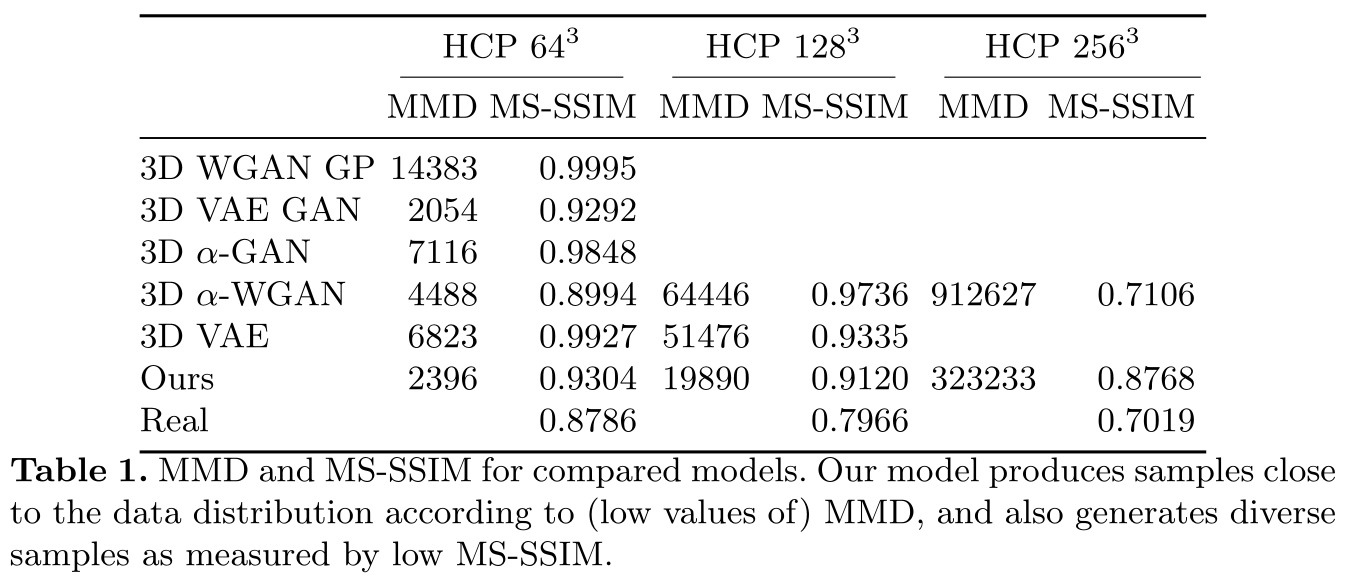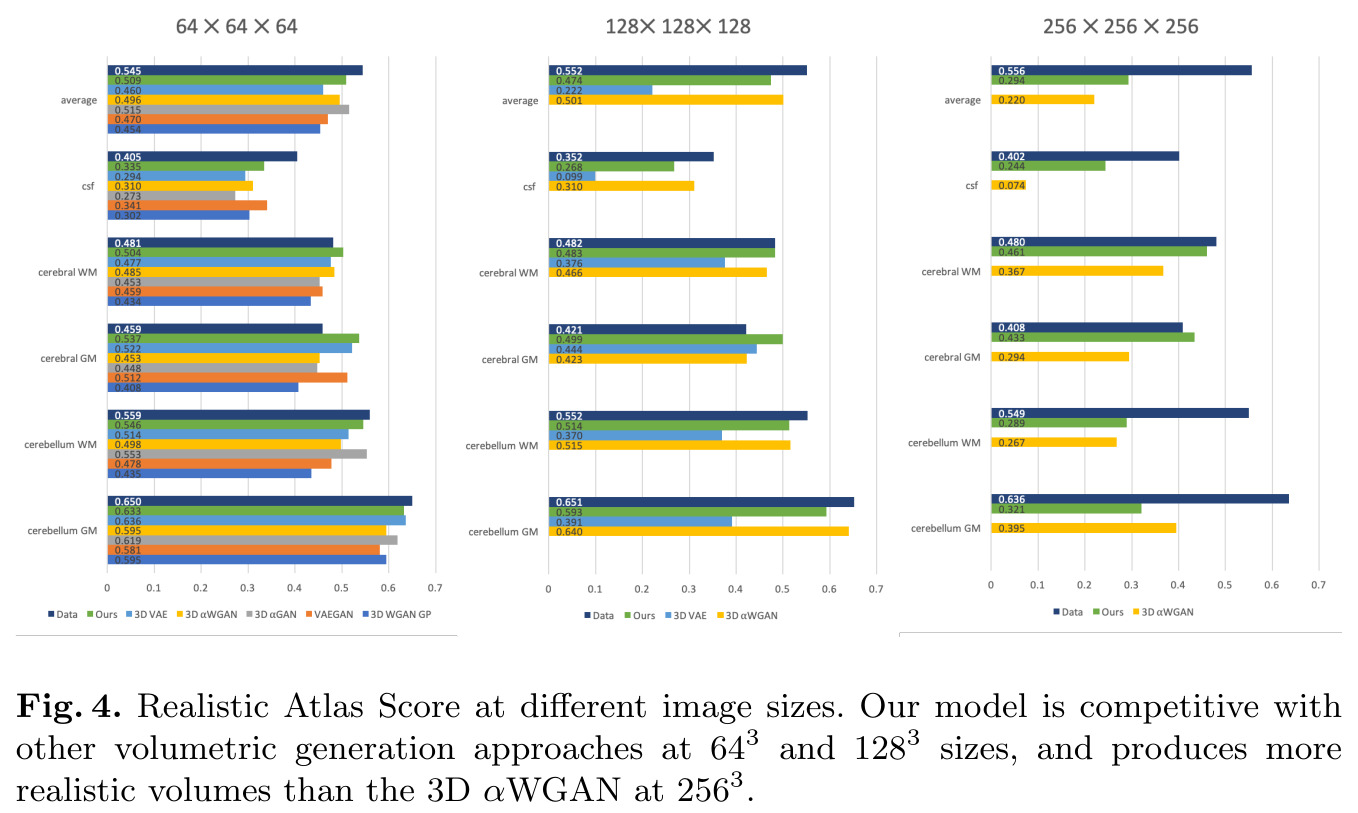# Highlights

• Method to model 3D MR brain volumes distribution by combining a 2D slice VAE with a Gaussian model that captures the relationships between slices;
• Novel evaluation method for generated volumes that quantifies how well their segmentations match those of true brain anatomy, called the Realistic Atlas Score (RAS).

# Introduction

Although Variatonal Autoencoder (VAE) models have become popular in the last few years to model the distribution of 2D data, their application to 3D data remains very limited. This can be explained by limitations in available training data and computational resources. Thus, volumetric VAEs mainly showed results on down-sampled coarse 3D data, for example 3x64x64x32 space-time cuboids, instead of the more usual 256x256x256 resolution.

# Methods

## Modeling distribution of 3D images with 2D VAE

The authors’ proposed method is quite simple. They train a 2D VAE to generate MRI slices, and use a Gaussian model to represent the distribution of each latent variable across the slices. Latent variables are assumed to be independent.Thus, the Gaussian model for the latent vectors is:

$p(\mathbf{y}_l) = \mathcal{N}(\mathbf{y}_l | \mathbf{\mu}_l,\mathbf{\Sigma}_l), \mathbf{y}_l = [y_l(1),\dots,y_l(t),\dots,y_l(T)]$

where $$y_l(t)$$ is the $$l$$-th component of the latent vector at slice $$t$$.

## Evaluating quality of the generated samples with RAS

The authors also propose a new metric to evaluate how “real” a generated volume appears to both a segmentation network and a registration algorithm. Their metric can be computed like follows:

1. Segment the generated samples using a segmentation network trained using real images;
2. Register the generated samples to real images and map the predicted segmentation with the same transformation.
3. Evaluate the Dice score on the generated samples’ registered segmentations.

# Data

The authors apply their method to T1 weighted MRI volumes from the Human Connectome Project (HCP) dataset, with some custom preprocessing. In the end, they use 960 volumes for training and 40 for validation, resampled to ensure a consistent size of 256x256x256 at 0.7x0.7x.07 mm3 resolution.

# Results# Remarks

• The authors often mention the “slice dimension”, but their VAE has not explicit notion of a “slice dimension”. Thus, the reliance on there being an interpretable “slice dimension” is optimist;